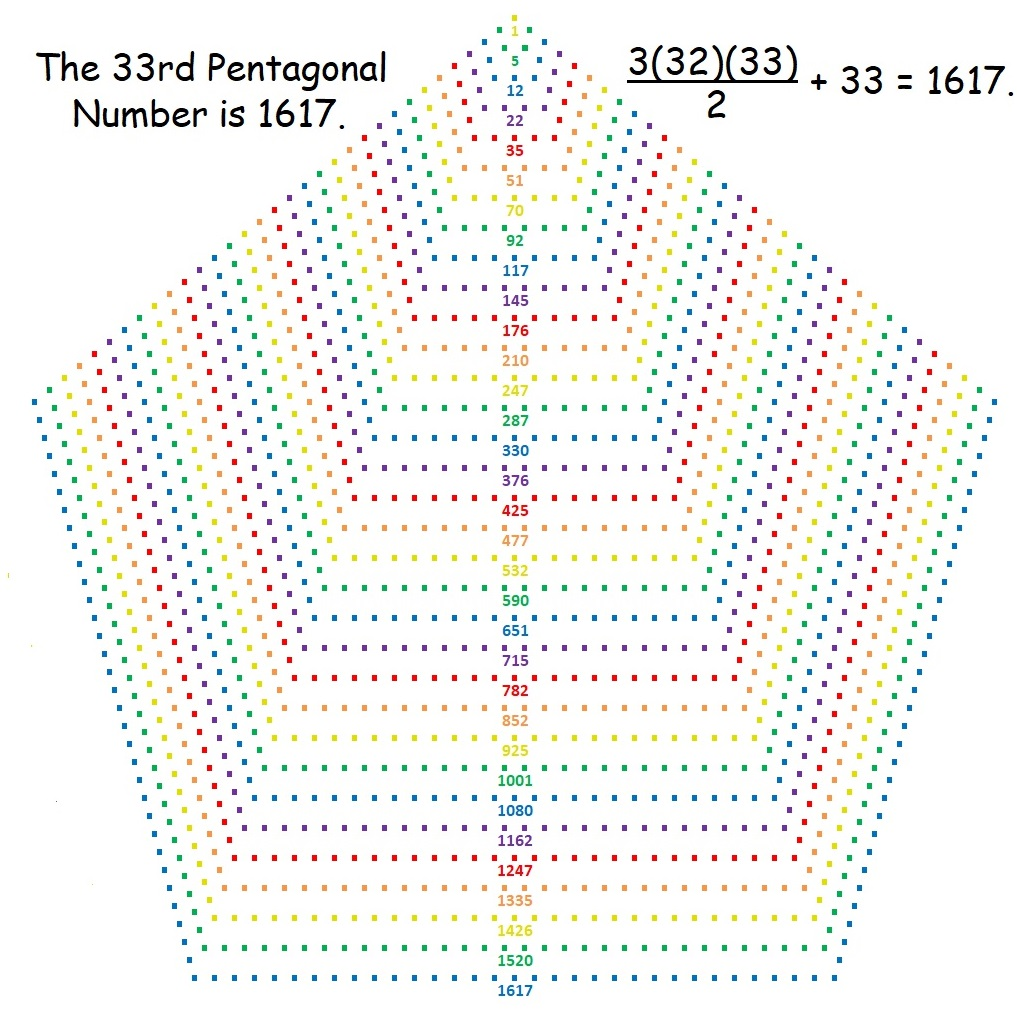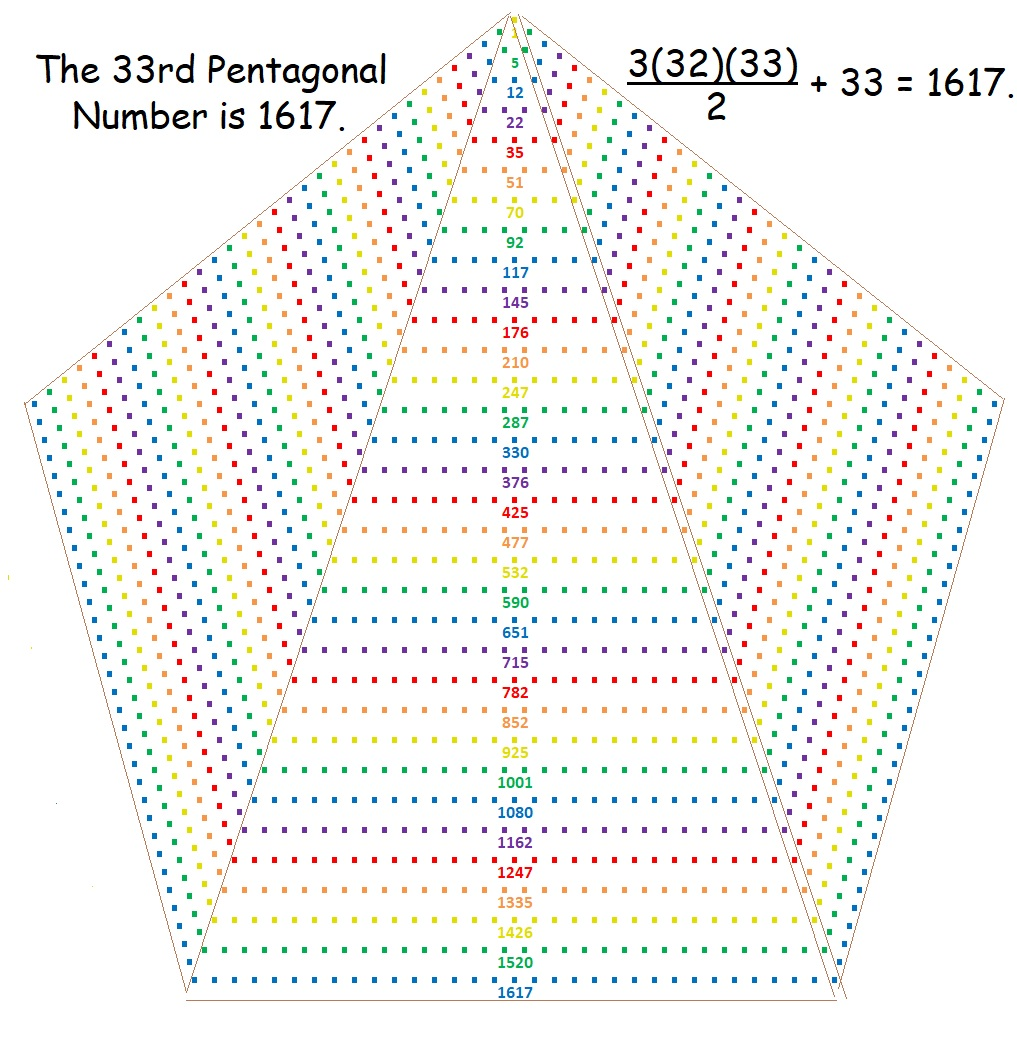1617 is a Pentagonal Number

Contents

Today’s Puzzle:

1617 is the 33rd pentagonal number because it is the 33 more than three times the 32nd triangular number. You can see the formula for that in the graphic below.

Can you draw lines to form three triangles each with 32 dots on its base and have exactly 33 dots left over? There are several different ways to solve this puzzle. You can scroll down to see one of those ways.Factors of 1617:

1 + 1 + 7 = 9, a number divisible by 3, so 1617 is divisible by 3. (It wasn’t necessary to add the 6 because we already know 6 is divisible by 3.)
1 – 6 + 1 – 7 = -11, so 1617 is divisible by 11.

• 1617 is a composite number.
• Prime factorization: 1617 = 3 × 7 × 7 × 11, which can be written 1617 = 3 × 7² × 11.
• 1617 has at least one exponent greater than 1 in its prime factorization so √1617 can be simplified. Taking the factor pair from the factor pair table below with the largest square number factor, we get √1617 = (√49)(√33) = 7√33.
• The exponents in the prime factorization are 1, 2, and 1. Adding one to each exponent and multiplying we get (1 + 1)(2 + 1)(1 + 1) = 2 × 3 × 2 = 12. Therefore 1617 has exactly 12 factors.
• The factors of 1617 are outlined with their factor pair partners in the graphic below.1617 is the difference of two squares in SIX different ways:
809² – 808² = 1617,
271² – 268² = 1617,
119² – 112² = 1617,
79² – 68² = 1617,
49² – 28² = 1617, and
41² – 8² = 1617.

One Solution to the Puzzle:

Here is one of several possible solutions to the puzzle. Click on it if you want to see it more clearly.This site uses Akismet to reduce spam. Learn how your comment data is processed.